## stochastic effects in magnetic recording

In previous posts, we discussed magnetic recording. Here, we extend this discussion by looking at the effect of a thermal bath that is coupled to magnetic moments during recording.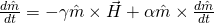where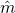is an unit vector describing the orientation of the magnetic moment,is time,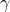is the gyromagnetic ratio,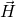is the effective field and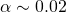is a damping constant. Landau added the second term in order that the magnetization moment would come into equilibrium (e.g.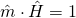and therefore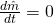) eventually. The equation has been derived in multiple theories for particular cases. In particular,  recovered LLG from a quantum macrospin in the limit of constant coupling and uniform density of states of the thermal bath.

For small magnetic particles, Brown  derived the required stochastic field from the Fokker-Planck equation. The resulting stochastic field,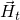, is a Gaussian with mean of zero and variance,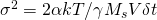in each dimension where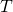is temperature,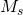is the saturation magnetization and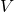is the volume of the magnetic grain.

The stochastic field will cause a diffusion offrom its equilibrium. Then, since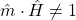the precession term will introduce a rotational flux of. Ignoring this rotational flux, we can define a diffusion coefficient of the magnetization as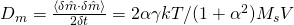where the last equality is calculated by replacingwith Brown’s stochastic fieldsin the LLG and integrating over all. Reasonable values would give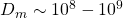rad/s. Roughly speaking this would mean in 1nswould have diffused ~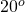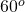from equilibrium. This does not include the additional processional term which also depend on this angle.

Instead of diffusion of magnetization, one could look at diffusion of free energy,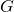, of the magnetic moment. In this case,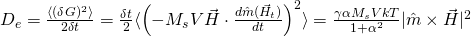and one can show Einstein’s relation holds,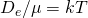, where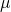is advective flux due to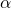in the absence of stochastic fields, namely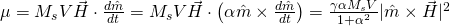.

From above, any analysis should includesince it’s a consequence of damping (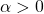). We will show its effect during conventional magnetic recording.

For conventional writing, as discussed previously, the applied write field goes as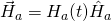such that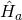is a slowly varying function of time (i.e. medium motion). On the other hand,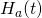is quickly varying in ~ 0.1ns with a peak large enough to eliminate the free energy barrier (e.g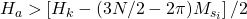). Therefore, one would expect the diffusive flux (e.g.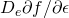) would lower required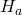.

We first confirmdoes, in fact, result in a Boltzmann distribution of the orientation of.

For micro-magnetic simulations, we have identical square prisms (extruded 10nm) grains of volume 503nmpacked in a periodic square lattice with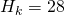kOe,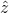(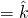) is normal to media plane,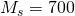emu/cc and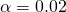.

For simplicity, we take 65536 grains,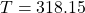K and exclude applied and magnetostatic fields. We start with all the grains alongand integrate in time until equilibrium is achieved.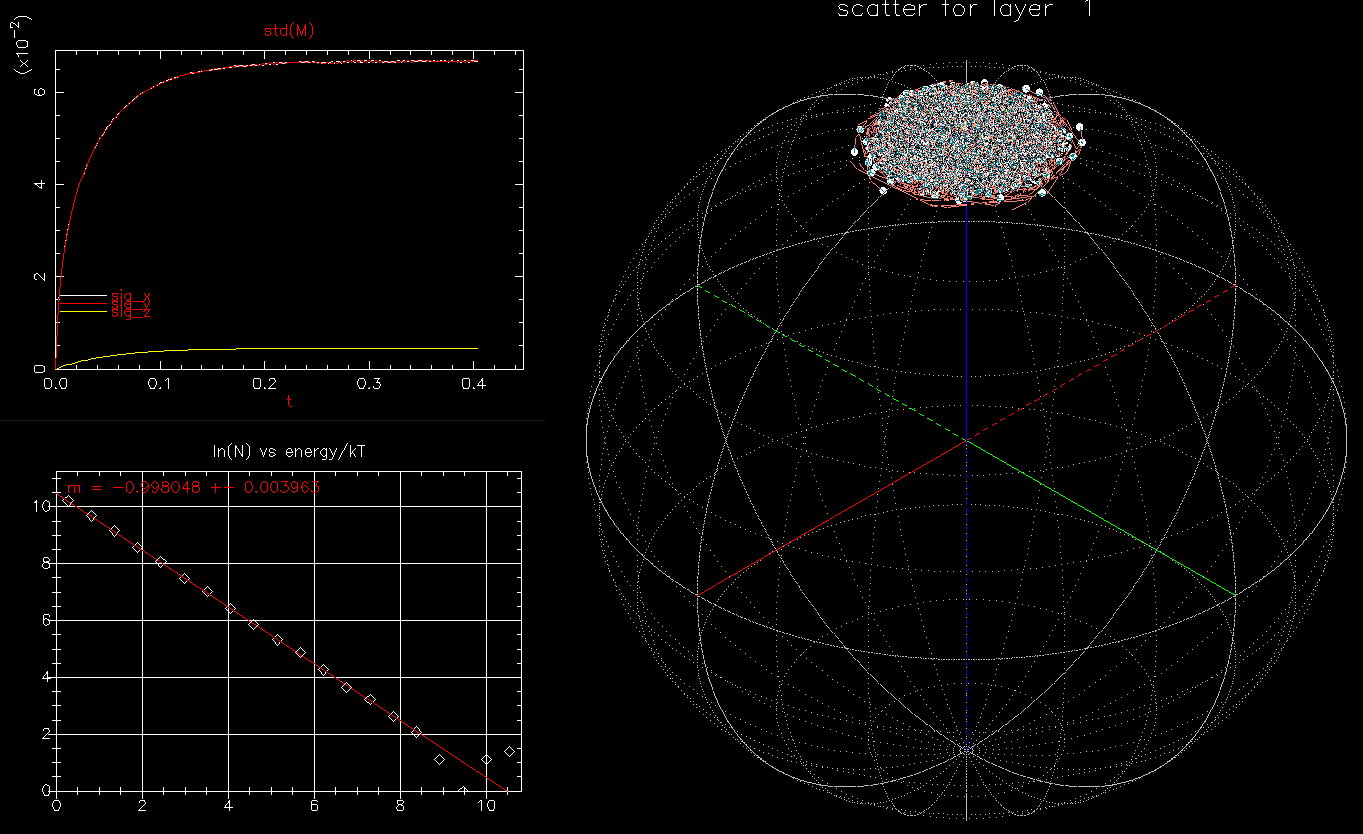65,536 identical grains subject to stochastic fields and . Upper left, standard deviation of and as a function of time (ns); lower left, histogram of as a function of energy; right, isometric projection of all grain orientations on a unit sphere: each white circle represents one magnetic orientation with orange trail showing short time history of orientation.

We see not only is the standard deviation ofis as expected at equilibrium but the time evolution to equilibrium is also correct. Further, the distribution ofas a function of energy is, in fact, a Boltzmann distribution (thereby ensuring the diffusive and drift flux will exactly balance). Of course, without, the variance would be identically zero, the distribution function would be a delta function at zero energy and the phase space plot would show all 65,536 grain orientations at the north pole (i.e.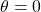).

We next use 1024 identical square prism grains with full magnetostatic fields and apply an uniform field of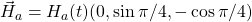linearly increasingfrom zero toin 0.1ns. For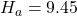kOe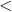9.6 kOe (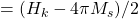) no grains should flip. We confirm this by running with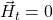: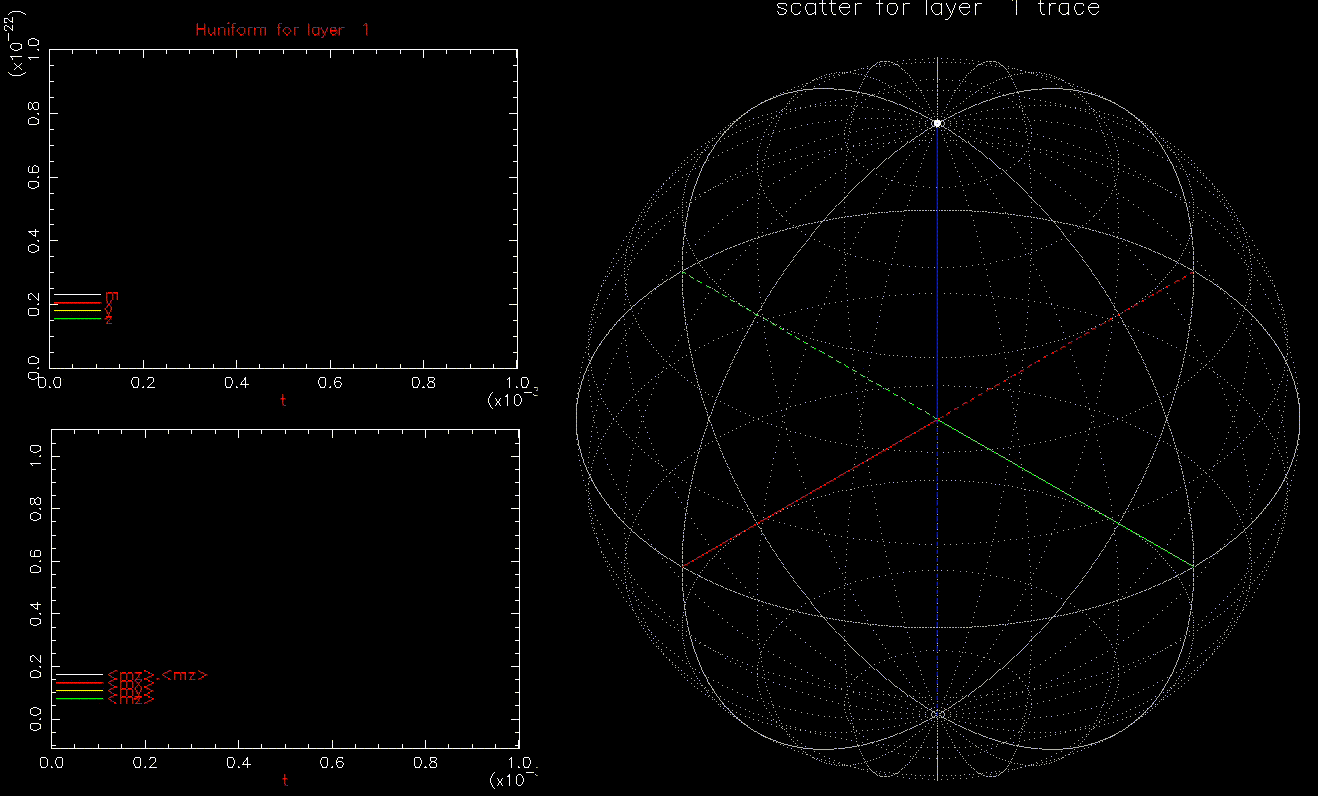Upper left: applied field (Oe) as a function of time (ns), white, red, yellow, green are magnitude, x, y, z components, respectively; bottom left: as a function of time (ns), white, red, yellow, green are magnitude, x, y, z components, respectively; right: isometric projection of unit sphere, dot represent the orientation of one of the 1024 grains updated every 1ps with line connecting to previous orientation. and is ramped from 0 to 9.45 kOe in 0.1ns, full magnetostatic field and .

We use the same identical set up, but now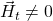: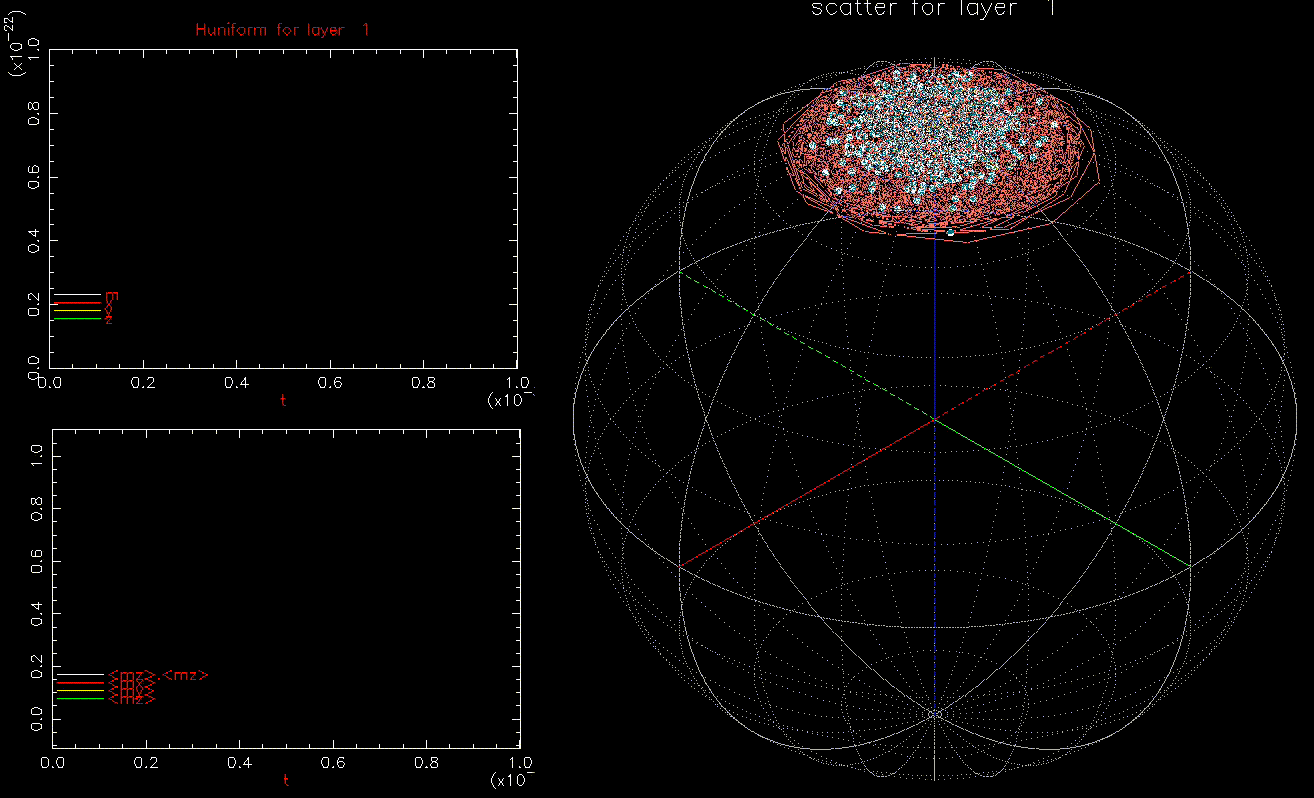Upper left: applied field (Oe) as a function of time (ns), white, red, yellow, green are magnitude, x, y, z components, respectively; bottom left: as a function of time (ns), white, red, yellow, green are magnitude, x, y, z components, respectively; right: isometric projection of unit sphere, dot represent the orientation of one of the 1024 grains updated every 1ps with line connecting to previous orientation, with line reset every 50ps. and is ramped from 0 to 9.45 kOe in 0.1ns, full magnetostatic field and . At 1ns, 62% of the grains have switched (not shown).

We also note that in the absence of,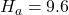kOe was sufficient to flip all 1024 grains. However, sincebreaks the symmetry, one requires~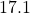kOe to switch all grains in 1ns. Therefore we see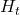does lowerfor both first grain flip (~ 7kOe) and all grains flip.

For completeness, we repeat the previous calculations but we rampin 0.1ns (instead of 5ns) and include:Required when is ramped from 0 to in 0.1ns and kept there for an additional 0.5ns. Values in ‘[]‘ are with and are dependent on the time is held constant.

The one clear conclusion we see is that the ‘switching field distribution’ (the difference betweenfor switching all grains to switching one grain) increases when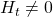. This is usually due to the reduction ofneeded to switch the first grain. When, the results above depend on the time thatis applied. For longer times (0.5ns used here),would decrease.

This, once again, shows the importance of including the full magnetostatic and the stochastic fields during conventional writing. Be aware that many simulations reported in the literature don’t included either one or both (i.e. “effective write field” for switching)- they will lead you to wrong conclusions if you’re trying to design a new storage system.

 A. Rebei and G.J. Parker, Fluctuations and dissipation of coherent magnetizationPhys. Rev. B67, 104434, (2003).

 W.F. Brown, Thermal fluctuations of a single-domain particle, Phys. Rev., 130, 1677 (1963).